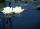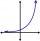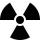Sum-log

The sum of two numbers is 32, the sum of their logarithms (base 10) is 2.2. Determine these numbers.

Result

x =  25.875
y =  6.125

Solution:Leave us a comment of this math problem and its solution (i.e. if it is still somewhat unclear...):Be the first to comment!To solve this verbal math problem are needed these knowledge from mathematics:

Looking for help with calculating roots of a quadratic equation? Do you have a linear equation or system of equations and looking for its solution? Or do you have quadratic equation?

Next similar math problems:

1. Exponential equationSolve exponential equation (in real numbers): 98x-2=9
2. Water liliesWater lilies are growing on the pond and their number is doubled every day. The whole layer is covered in 12 days. How many days will it cover 8 layers?
3. Ball gameRichard, Denis and Denise together scored 932 goals. Denis scored 4 goals over Denise but Denis scored 24 goals less than Richard. Determine the number of goals for each player.
4. Theorem proveWe want to prove the sentence: If the natural number n is divisible by six, then n is divisible by three. From what assumption we started?
5. DiscriminantDetermine the discriminant of the equation: ?
6. RootsDetermine the quadratic equation absolute coefficient q, that the equation has a real double root and the root x calculate: ?
7. EquationEquation ? has one root x1 = 8. Determine the coefficient b and the second root x2.
8. Intercept with axisF(x)=log(x+4)-2, what is the x intercept
9. Powers 32 to the power of n divided by 4 to the power of -3 equal 4. What is the vaule of n?
10. Exponential equationIn the set R solve the equation: ?After 548 hours decreases the activity of a radioactive substance to 1/9 of the initial value. What is the half-life of the substance?Solve quadratic equation: (6n+1) (4n-1) = 3n2Find the roots of the quadratic equation: 3x2-4x + (-4) = 0.Quadratic equation ? has roots x1 = -26 and x2 = -86. Calculate the coefficients b and c.The group has 42 children. There are 4 more boys than girls. How many boys and girls are in the group?How many real roots has equation ? ?Solve two equations with two unknowns: 400x+120y=147.2 350x+200y=144